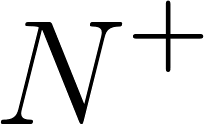×
Get Full Access to A Transition To Advanced Mathematics - 7 Edition - Chapter 5.3 - Problem 1
Get Full Access to A Transition To Advanced Mathematics - 7 Edition - Chapter 5.3 - Problem 1

×

# What is the 28th term in the sequence of positive rationals produced by thecountingISBN: 9780495562023 335

## Solution for problem 1 Chapter 5.3

A Transition to Advanced Mathematics | 7th Edition

• Textbook Solutions
• 2901 Step-by-step solutions solved by professors and subject experts
• Get 24/7 help from StudySoup virtual teaching assistantsA Transition to Advanced Mathematics | 7th Edition

4 5 1 317 Reviews
18
2
Problem 1

What is the 28th term in the sequence of positive rationals produced by thecounting process described in the discussion of Theorem 5.3.1?

Step-by-Step Solution:

Problem 1

What is the 28th term in the sequence of positive rationals produced by the counting process described in the discussion of Theorem 5.3.1?

Step by Step Solution

Step 1 of 2

The sequence formed by taking elements from diagonals above pattern defines a one to one correspondence fromThe sequence is the function itself.

Therefore find theterm of the sequence.

Step 2 of 2

##### ISBN: 9780495562023

This textbook survival guide was created for the textbook: A Transition to Advanced Mathematics, edition: 7. This full solution covers the following key subjects: . This expansive textbook survival guide covers 39 chapters, and 619 solutions. The answer to “What is the 28th term in the sequence of positive rationals produced by thecounting process described in the discussion of Theorem 5.3.1?” is broken down into a number of easy to follow steps, and 22 words. Since the solution to 1 from 5.3 chapter was answered, more than 323 students have viewed the full step-by-step answer. A Transition to Advanced Mathematics was written by and is associated to the ISBN: 9780495562023. The full step-by-step solution to problem: 1 from chapter: 5.3 was answered by , our top Math solution expert on 03/05/18, 08:54PM.

Unlock Textbook Solution